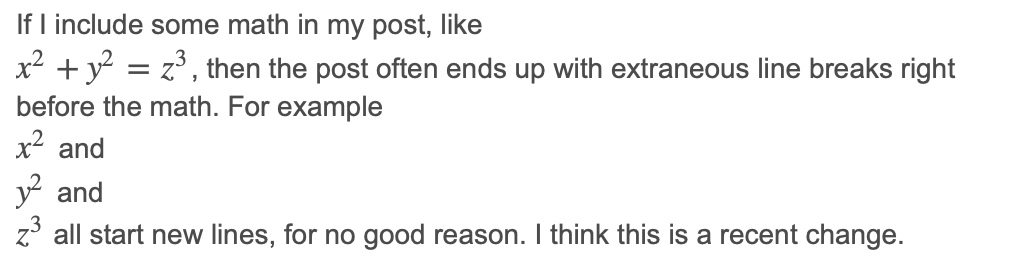# Revision history [back]

### Math typesetting partly broken

If I include some math in my post, like $x^2 + y^2 = z^3$, then the post often ends up with extraneous line breaks right before the math. For example $x^2$ and $y^2$ and $z^3$ all start new lines, for no good reason. I think this is a recent change.

### Math typesetting partly broken

If I include some math in my post, like $x^2 + y^2 = z^3$, then the post often ends up with extraneous line breaks right before the math. For example $x^2$ and $y^2$ and $z^3$ all start new lines, for no good reason. I think this is a recent change.

### Math typesetting partly broken

If I include some math in my post, like $x^2 + y^2 = z^3$, then the post often ends up with extraneous line breaks right before the math. For example $x^2$ and $y^2$ and $z^3$ all start new lines, for no good reason. I think this is a recent change.

### Math typesetting partly brokenbroken on Safari

If I include some math in my post, like $x^2 + y^2 = z^3$, then the post often ends up with extraneous line breaks right before the math. For example $x^2$ and $y^2$ and $z^3$ all start new lines, for no good reason. I think this is a recent change.

Here is a screenshot:5 None

### Math typesetting partly broken on Safari

If I include some math, like $x^2 + y^2 = z^3$, in an Ask Sage question or answer, then the post often ends up with an extraneous line break right before each inline math formula, when viewed in Safari on macOS.

For example $x^2$ and $y^2$ and $z^3$ all start new lines, for no good reason. I think this is a recent change.

Here is an earlier version of this same question:

If I include some math in my post, like $x^2 + y^2 = z^3$, z^3$, then the post often ends up with extraneous line breaks breaks right before the math. For example$x^2$and$y^2y^2$and$z^3\$ all start new lines, for no good reason. reason.
I think this is a recent change. Here is a screenshot:change.


and a screenshot of how it was rendered in Safari:A web search for [ mathjax safari newline ] reveals similar questions:

The MathJax issue link suggests that it's been fixed in a more recent MathJax version. Can we update the version of MathJax used on this site?